Friday, January 30, 2015

January 2015 Geometry Regents Parts II, II, IV (Open-Ended)

Once again, here are the multiple-choice problems with explanations for today's New York State Geometry Regents exam. Since I typed this up as quickly as I could, there are no images, graphs or diagrams included. I'll edit them as I am able to.

A common theme: there were several questions involving the equation of a circle, as there always are, as well as several questions which not only involved the Pythagorean Theorem, but very specifically a 3-4-5 triangle or a multiple of it.

I hope everyone did well. Here is are the (most of the) open-ended questions. Again, sorry, no images.

Part II

29. The Proof. Image to be uploaded later.

Very simply, you are given two pairs of congruent sides. The included angles, BCA and DCE are congruent because they are vertical angles. Therefore ABC is congruent to DEC by SAS.

30. Using a compass and straightedge, construct the perpendicular bisector of side AR in triangle ART show below. [Leave all construction marks.] (image omitted)

I can’t really show this, but keep in mind that point T is irrelevant. It is NOT on the bisector. Ignore the triangle and focus on segment AR. Create the perpendicular bisector as you would with any other segment.

31. Determine and state the measure, in degrees, of an interior angle of a regular decagon.

First, even if you have this memorized, you still need to show your work.

Second, there are two methods, both of which are acceptable.

Interior:  (10 – 2) * 180 = 1440. 1440 / 10 = 144 degrees for each interior angle.

Or exterior: 360/10 = 36 degrees per exterior angle. Interior angle = 180 – 36 = 144 degrees.

32. Write an equation of a line that is parallel to the line whose equation I s 3y = x + 6 and that passes through the point (-3, 4).

Note that it says “an equation” and doesn’t say that it has to be in any specific form.

Easiest method, plug in (-3, 4) into the equation and find the new constant. (I won’t call it “b”.)

3(4) = -3 + c

12 = -3 + c

15 = c

3y = x + 15 is an acceptable answer

In slope-intercept form, it is y = 1/3x + 5.

33. In the diagram below, secants PQR and PST are drawn to a circle from point P. (image omitted)

If PR = 24, PQ = 6, and PS = 8, determine and state the length of PT.

Not a trick question. No extra adding or subtracting.

(PQ)(PR) = (PS)(PT)

(6)(24) = (8)(x)

144 = 8x

18 = x

PT is 18.

34. The slope of QR is (x -1)/4 and the slope of ST is 8/3. If QR is perpendicular to ST, determine and state the value of x.

If QR is perpendicular to ST, then the slope of QR is -3/8. So

(x-1)/4 = -3/8

8(x-1) = -12

8x – 8 = -12

8x = -4

X = -1/2

Part III

35. Quadrilateral HYPE have vertices H(2,3), Y(1, 7), P(-2, 7) and E(-2, 4). State and label the coordinates of the vertices of H’’Y’’P’’E’’ after the composition of transformations r x-axis o T 5, -3.

Remember: the translation comes before the reflection. If you do it the other way around, you will lose half-credit (2 points). You MUST label the coordinates of the final points. Keep this in mind if you are using the graph!

H(2, 3) > H’(7, 0) > H’’(7, 0)

Y(1, 7) > Y’(6, 4) > Y’’(6, -4)

P(-2, 7) > P’(3, 4) > P’’(3, -4)

E(-2, 4) > E’(3, 1) > E’’(3, -1)

36. On the set of axes below, graph two horizontal lines whose y-intercepts are (0, -2) and (0, 6), respectively.

Graph the locus of points equidistant from these horizontal lines.

Graph the locus of points 3 units from the y-axis.

State the coordinates of the points that satisfy both loci.

The first locus is the horizontal line y = 2.

The second locus is comprised of the vertical lines x = -3 and x = 3.

The coordinates of the points that satisfy both conditions are (-3, 2) and (3, 2).

37. In the diagram below, a right circular cone with a radius of 3 inches has a slant height of 5 inches and a right cylinder with a radius of 4 inches has a height of 6 inches.

(image omitted)

Determine and state the number of full cones of water needed to completely fill the cylinder with water.

You need to know the Volume of each solid. You can leave them in terms of pi.

First, you need to know the height of the cone – you were given the slant height.

Notice that one more time the problem involves a 3-4-5 right triangle. Satisfy yourself however you have to that the height of the cone is 4 inches.  (State that it’s a right triangle with dimensions 3-4-5, using Pythagorean Theorem.)

The cone has V = 1/3 (pi) r2 h = 1/3 (pi) (9) (4) = 12 pi

The cylinder has V = (pi) r2 h = (pi) (16)(6) = 96 pi.

Divide 96pi/12pi = 8. (Pi will cancel out.)

8 cones will fill up the cylinder.

Part IV

38. In the diagram below, right triangle RSU is inscribed in circle O, and UT is the altitude drawn to hypotenuse RS. The length of RT is 16 more than the length of TS and TU = 15.

Find the length of TS.

Find, in simplest radical form, the length of RU.

(Image omitted)

You need to use the Right Triangle Altitude Theorem, and Pythagorean Theorem. And then simplify the radicals.

First part: x / 15 = 15 / x + 16

Cross-multiply: x2 + 16x = 225

So x2 + 16x – 225 = 0

(x + 25)(x – 9) = 0

X = -25 or x = 9.

This makes TR = 9 + 16 = 25.

RU = the square root of (252 + 152) = the square root of (625 + 225) = the square root of (850).

The largest perfect square that is a factor of 850 is 25, which goes into 850 34 times.

RU = (25).5(34).5 = 5(34).5. (That is, 5 times the square root of 34.)

Yes, that was a crazy problem, but gettable if you worked your way through it.

For instance, you can find the length of US, which is the square root of 306. And the square root of (306 + 850) = square root of 1156 = 34, which is the length of the diameter of circle O. We know this because ST is 9 and TR is 25, and 9 + 25 = 34.

Thirty

(Click on the comic if you can't see the full image.)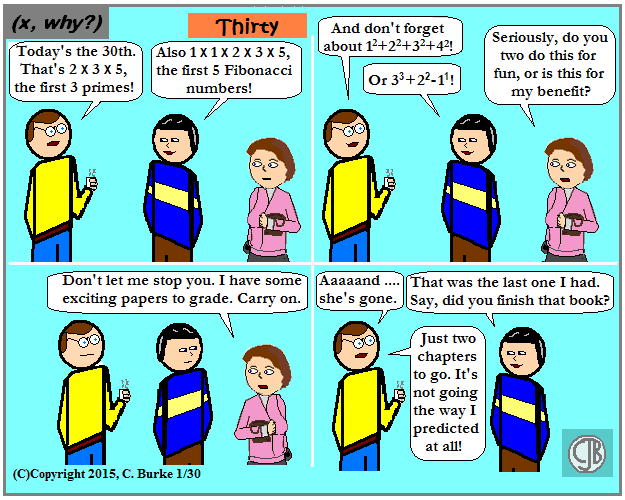Fun fact: if I didn't post this today, I'd have to wait two more months to use it.

By tradition, "30" also means the end of a news story even though more months end in 31 days. That's because it has nothing to do with the end of the month and more to do with the letters XXX, which is 30 in Roman numerals.

Not that there's any hidden meaning here. This is not the end of anything.

... except for these notes, of course.Wednesday, January 28, 2015

January 2015 Geometry Regents exam, Multiple-Choice

Once again, here are the multiple-choice problems with explanations for today's New York State Geometry Regents exam. The open-ended problems will (most likely) appear tomorrow. Since I typed this up as quickly as I could, there are no images, graphs or diagrams included. I'll edit them as I am able to.

A common theme: there were several questions involving the equation of a circle, as there always are, as well as several questions which not only involved the Pythagorean Theorem, but very specifically a 3-4-5 triangle or a multiple of it.

I hope everyone did well. Part I was, in my opinion, very "get-able" -- not very difficult for a Regents exam.

As always, apologies in advance for typos. I did rush this a bit. Here is Part I.

EDIT --- RESTORED from back-up

1.  What is the solution of the system of equations graphed below? (Image omitted)

y = 2x + 1

y = x2 + 2x – 3

The solutions of a system are the points where the two lines intersect. In this problem, that would be at (-2, -3) and (2, 5). Choice (4).

If you look at the other choices, (0, -3) is the y-intercept of the parabola, (-1, -4) is the vertex and (-3,0) and (1, 0) are the roots of the parabola. None of those have anything to do with the linear equation.

2. What are the coordinates of the midpoint of the line segment with endpoints (2, -5) and (8, 3)?

Take the average of the x-coordinates and of the y-coordinates.  You get (5, -1) which is exactly in the middle. Alternatively, you could have used the scratch graph paper and plotted it instead of calculating.

3.  As shown in the diagram below, when hexagon ABCDEF is reflected over line m, the image is hexagon A’B’C’D’E’F’. Under this transformation, which property is not preserved?

Orientation is not preserved. The object has the same shape and size, but the direction its facing switches.

4.  In the diagram of triangle ABC below, BD is drawn to side AC.

If m<A = 35, m<ABD = 25, and m<C = 60, which type of triangle is BCD?

BCD is equilateral. Angles A and ABD add up to 60 degrees. This makes <ADB = 120 degrees. Angle BDC is supplementary to ADB, so it is 60 degrees. Angle C is 60 degrees as well, so <CBD must be 60 degrees. Triangle BCD has three 60-degree angles, so it is an equilateral triangle.

5. In the diagram below of rhombus ABCD, the diagonals AC and BD intersect at E. (Image omitted)

If AC = 18 and BD = 24, what is the length of one side of rhombus ABCD?

The diagonals of a rhombus are perpendicular and bisect each other. They form four congruent right triangles. Each side of the rhombus is the hypotenuse of one of those right triangles. AC = 18, so AE = 9. BD = 24, so BE = 12. If the two legs of a right triangle are 9 and 12, then the hypotenuse is 15. Note: You can use the Pythagorean Theorem, but you should know 9-12-15 because it’s a multiple of 3-4-5, which is the most common right triangle used in these problems! The answer is 15.

6. What are the truth values of the statement “Opposites angles of a trapezoid are always congruent.” and its negation?

The statement is false, which makes the negation (“Opposite angles of a trapezoid are not always congruent.” ) true.

7. What is the length of a line segment whose endpoints have coordinates (5, 3) and (1, 6)?

Use the distance formula, or sketch the line segment, make a right triangle and use Pythagorean Theorem. Either way, you will get the square root of (32 + 42), which is the square of (9 + 16), which is 5. Again, 3-4-5 is a common right triangle. Recognize it when you see it!

8.  In the diagram below of isosceles triangle ABC, the measures of vertex angle B is 80 degrees. If AC extends to point D, what is m<BCD?

The vertex angle is 80 degrees, so the two base angles have a total of 100 degrees. The base angles are congruent, so each is 50 degrees.  Angle BCD is supplementary to a 50 degree angle, so it is 130 degrees.

9. A student used a compass and a straightedge to construct CE in triangle ABC as shown below.

Which statement must always be true for this construction?

The construction shows the bisecting of angle C. So <ACE is congruent to < BCE.

10. In triangle, ABC, AB = 4, BC = 7, and AC = 10. Which statement is true?

The smallest angle is across from the smallest side and the biggest angle is across from the biggest side. (Triangle Inequality Theorem). The opposite angle will be the vertex that is NOT part of the line segment; i.e., <C is across from AB and is the smallest angle, and <B is the largest.  The correct choice is (2) m<B > m <A > m< C.  (Sorry about the formatting. It can’t be helped.)

11. A circle whose center has coordinates (-3, 4) passes through the origin. What is the equation of this circle?

Using Pythagorean Theorem, we can see that the radius of the circle is 5. Again, another 3-4-5 triangle!

Flip the signs and square the radius: (x + 3)2 + (y – 4)2 = 25

12. Point W is located in plane R. How many distinct lines passing through point W are perpendicular to plane R?

One.  Think of any vertical pole coming out of the ground. There’s only one possible vertical pole at that point in the ground.

13. In the diagram below, line l is parallel to line m, and line w is a transversal.

If m<2 = 3x + 17 and m <3 = 5x – 21, what is m<1?

Angles 2 and 3 are supplementary. Angles 1 and 2 are congruent. Solve for x and then find the measure of angle 2 to get the answer.

3x + 17 + 5x – 21 = 180

8x – 4 = 180

8x = 184

x = 23

m<2 = 3(23) + 17 = 69 + 17 = 86. Choice (4).

Note: be careful. I subtracted 4 instead of adding first time through. My incorrect answer wasn’t one of the choices, so I knew to go back and check my work!

14. The diagram below is of circle O.

(A circle with center O at (5, -3) and a radius of 4.)

Which equation represents circle )?

Again. Flip the signs and square the radius. (x – 5)2 + (y + 3)2 = 16.

15. In isosceles trapezoid QRST show below, QR and TS are bases.

If m<Q = 5x + 3 and m<R = 7x – 15, what is m<Q?

Angles Q and R are congruent, so their measures are equal.

5x + 3 = 7x – 15

3 = 2x – 15

18 = 2x

9 = x

m<Q = 5(9)  + 3 = 45 + 3 = 48. Choice (2).

16. Triangle ABC is graphed on the set of axes below.

What are the coordinates of the point of intersection of the medians of triangle ABC?

This one is easier than it sounds. The median from vertex A to the midpoint of BC is a horizontal line with a length of 6. The medians will meet at the centroid, which is 2/3 of the way from A to the midpoint. That means that it will be 4 units to the right of point A. The answer is (-1, 2).

If you drew the three medians (two would be enough, though), you could probably eyeball the correct answer from the choices given.  Only one really makes sense.

17. Given the statement, “If a number has exactly two factors, it is a prime number,” what is the contrapositive of this statement?

Negative both parts and flip it around. “If a number is not a prime number, it does not have exactly two factors.”  Choice (2).

18. Which graph represents a circle whose equation is (x – 2)2 + (y + 4)2 = 4. (Images omitted)

Third time! Flip the signs. The center is (2, -4). The radius is 2, which is the square root of 4. That makes Choice (3) the correct answer.

19. If two sides of a triangle have lengths of 4 and 10, the third side could be …

The third side must be greater than 6, which is 10-4, and less than 14, which is 10+4. The only choice that fits is Choice (1) 8.

20. The lines represented by the equations 4x + 6y = 6 and y = 2/3x – 1 are …

Put the first line into slope-intercept form:

4x + 6y = 6

6y = -4x + 6

y = -2/3x + 1

The lines have the different slope, so they are neither parallel nor the same line. Additionally, the slopes are not negative reciprocals, so they are not perpendicular. However, they will intersect.

21. In the diagram below of triangle ABC, DE || AB.

If CD = 4, CA = 10, CE = x + 2 and EB = 4x – 7, what is the length of CE?

Set up a proportion. CD/DA = CE/EB.  Notice that I said DA, not CA. DA = 10-4 = 6.

So 4/6 = x+2/4x -7. Cross multiply add you get

4(4x – 7) = 6(x + 2)

16x – 28 = 6x + 12

10x = 40

X = 4

CE = 4 + 2 = 6. Choice (3).

22. Parallelogram ABCD with diagonals AC and BD intersecting at E is shown below.

Which statement must be true?

Diagonals of a parallelogram bisect each other, but that does not appear in the choices.

Each diagonal is a transversal across two parallel lines, so the alternate interior angles are congruent. This is needed to prove choice (2).

23. In the diagram below of circle O, m<ABC = 24.

What is m<AOC?

The inscribed angle is half the size of the central angle. If m<ABC is 24, then m<AOC = 48.

24. Triangle A’B’C’ is the image of triangle ABC after a dilation of 2. Which statement is true?

The original triangle will have sides that are half as long as the image. The angles will be the same. So the angle is choice (3), m<B = m<B’.

25. In the diagram of the circle below, AD || BC, arc AB = (5x + 30) degrees, and arc CD = (9x – 10) degrees.

What is mAB?

If the chords are parallel, then the arcs they create are congruent.

9x – 10 = 5x + 30

4x = 40

X = 10

AB = 5(10) + 30 = 50 + 30 = 80. Choice (4).

26. The bases of a prism are right trapezoids, as shown in the diagram below.

Which two edges do not lie in the same plane?

Choice (1), BC and WZ. You cannot find a piece of paper which lines up with both edges. They are skew.

27. In the diagram below, A’B’ is the image of AB under which single transformation?

It’s flipped over the x-axis and slid back a few spaces. It’s a glide reflection.

28. For which diagram is the statement triangle ABC ~ triangle ADE not always true?

Choice (4), which shows a trapezoid, with A as the midpoint of the diagonals. Triangles ABC and ADE share no angles and have none which must be congruent. Therefore, they are not always similar.

Saturday, January 24, 2015

Reviews of the Last 3.14 Book I Read

The title is a little misleading as there's no way that I made it anywhere near a seventh of the way through one of the books. However, in keeping up with a goal to read more and to write more, I've uploaded four reviews to my reading blog, which started out as simply someplace for me to keep track of what I've read and of the plots and characters of the more obscure ones.

Of the four books, all four were e-books, and only one of them I've ever actually seen in print. Two were fantasy, one sci-fi (time travel), and the last falls into the self-help category.

• Shards of the Glass Slipper: Queen Cinder by Roy A. Mauritsen, 2012.

• Dirty Machines by David Matthew Olson, 2014.

• 365 Things I Learned the Hard Way (So You Don't Have To) by The Digital Writer (Jonathan Wondrusch), 2012.

• The God in the Clear Rock by Lucian Randolph, 2011.

So check 'em out. (Well, maybe not that last one.)

• Friday, January 23, 2015

Name That Line!

(Click on the comic if you can't see the full image.)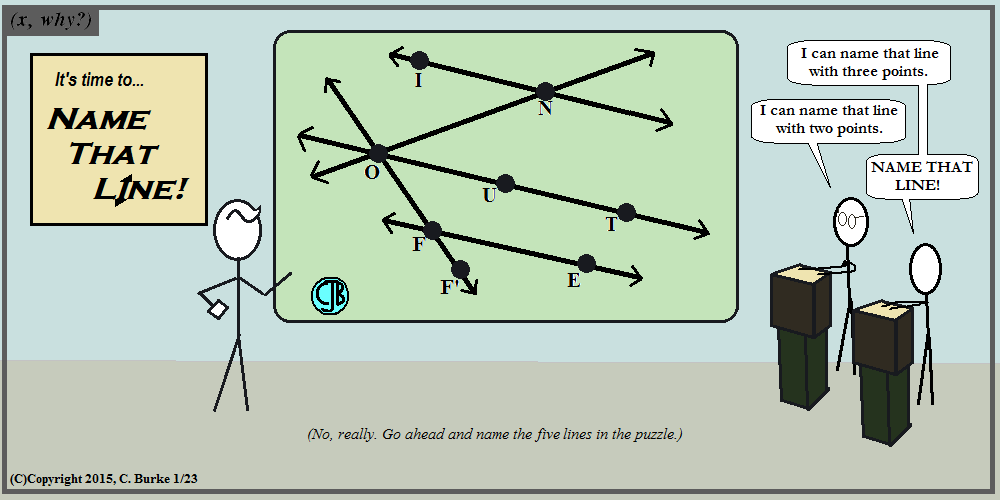I could have used the I to work in AIR and then JAW, but it was too much.

I don't know if that's Wink Martindale, Jim Lange, Dennis James or one of the other oldtime emcees.Wednesday, January 21, 2015

Pascals Triangle

(Click on the comic if you can't see the full image.)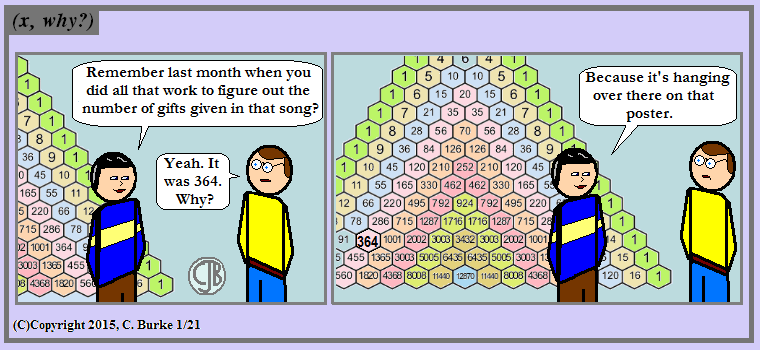This occurred to me a while ago, but after Christmas, and I decided that it couldn't wait until December. (And I'd likely forget about it.)

While coming up with mathematical formulas and computer code for calculating this number, I overlooked a a very reliable reference tool: Pascal's Triangle.

It has more uses than simply expanding polynomials because of its many properties.

Some of these properties are as follows:

• The "zeroth" element of each row is the number 1.
• The first element of each row is the number of the row (keeping in mind that the top row is Row 0).
• The second element of each row is are the consecutive triangle numbers, the sum of the consecutive numbers before it.
• Which makes the third element of each row the sum of the consecutive triangle numbers.
• And, finally, the position of each element in Pascal's Triangle corresponds to the number of Combinations designated by the notation nCr where n is the row and r is the element of that row.

What this means is that for any given day in that song (The Twelve Days of Christmas):

• nC1 refers to the day we're up to. On Day 7, 7C1 is 7.
• n+1C2 refers to the total number of gifts given on the nth day. On Day 7, 8C2 is 28.
• n+2C3 refers to the total number of gifts given altogether up to the nth day. On Day 7, 9C3 is 84.

Applying this to the 12th day of the song:

• 12C1 is 12.
• 13C2 is 78.
• 14C3 is 364.

And I could've been finished a whole lot sooner. But I wouldn't have gotten a recursive comic out of that.

One last thing: Did you ever try to make a poster of Pascal's Triangle? Have your students tried to do it for a math fair? The numbers start to get really big in the middle, really fast. (That's a post for another day.) But that's also why the poster in this comic is so large! Otherwise, it wouldn't be readable (and I had to modify the "364" so you could find it easily!).• Tuesday, January 20, 2015

(x, why?) Mini: Octonions

(Click on the comic if you can't see the full image.)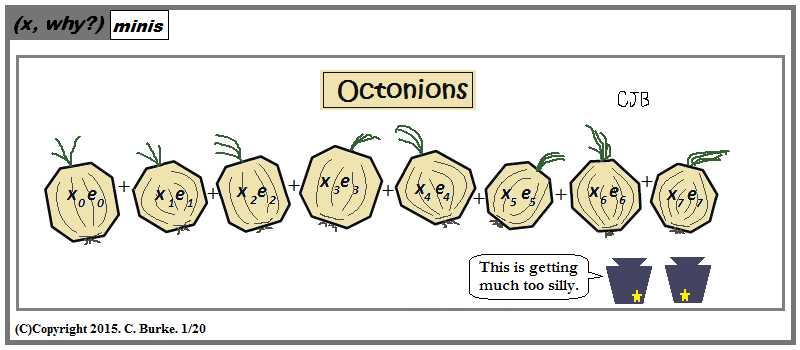Unfortunately, this is from a part of mathematics that has past me by. Something from after I started concentrating on my computer science studies in college.

I've seen the word "octonion" before, but it just popped up when i was lazily checking through a list of math blogs I bookmarked a couple years ago but haven't really visited. Sadly, I don't always know at first glance what they're talking about, and I rarely have the time to do the "catch-up" work to figure it out.Friday, January 16, 2015

Survey Says: Granularity!

(Click on the comic if you can't see the full image.)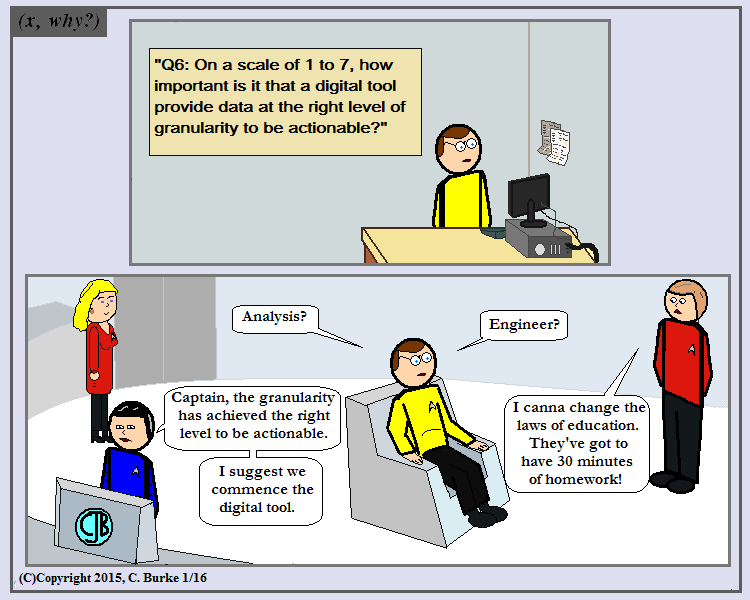All I had for Annie was "Cumulative Frequencies Open, Captain.", but it fit neither the space nor the flow of the dialogue.

True story. A very similar question appeared in an online survey, and that's what I imagined in my mind.

Oh, and the History teacher would've been the doctor if I wanted to extend the metaphor, but it was going too far already.Thursday, January 15, 2015

That Moment When . . . II

(Click on the comic if you can't see the full image.)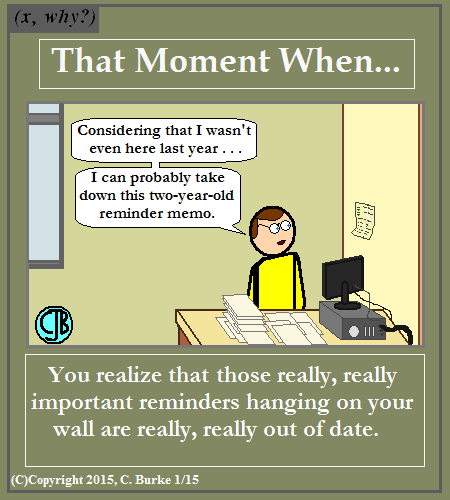At least most of the student work I'd hung up had been taken down. Most of it.

Another teacher, new to the school this year, asked me about document cameras, and wondered why she had the only math classroom without one. Equipment moves around sometimes, and she could request one. She told me a few days later that she happened to open the bottom drawer of her desk and found the camera in that drawer.

I stopped and stared in disbelief and told her the story. I was the first teacher to get one because the AP insisted I try it out. They were in such a hurry to set me up that they never locked it down or attached it to the desk or the computer. At the end of the year, after a brief though of larceny, I put it in that drawer so it would be out of sight and somewhat safe. Nearly a year and a half later, it was still in the same spot. Whoever had the room in the intervening time either hadn't used the drawer or didn't know how to use the camera.

By the way, this was the very same camera which was used to lure me into my surprise 50th birthday party.Tuesday, January 13, 2015

(x, why?) Mini: B for Bank?

(Click on the comic if you can't see the full image.)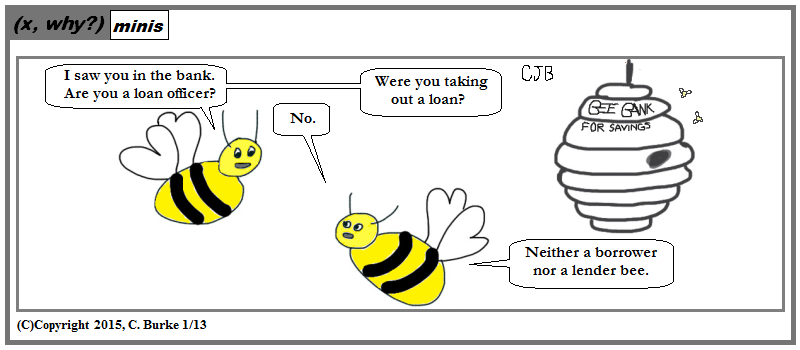Alas, so what then is the buzz?

(I bet you thought I was going to go with two bees or not two bees, didn't you? Not gonna do it.)Monday, January 12, 2015

Write? Check!

(Click on the comic if you can't see the full image.)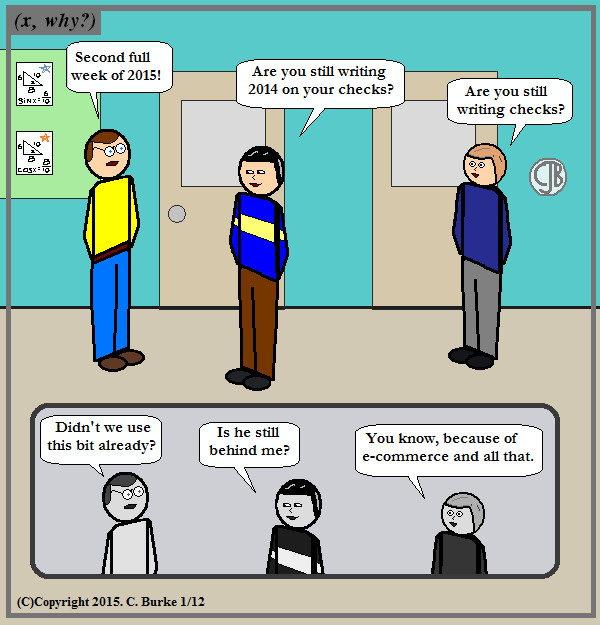Check? Write!

I still write a few, mostly for charitable organizations where it makes for a handy receipt.

What I really need to write is a name for the Science teacher! He doesn't have one. Almost all of the other (human) characters have names -- even Judy and Chuck, who were only named in a comic that neither of them were in, but were named nonetheless!

Maybe I should have a Contest. Or maybe a Suggestion Box. Does he look like a Tim? Gary? It shouldn't be anything too "out there" if only because Mike and Ken would've made fun of it by now (especially Ken). So what do you think?

In the meantime, write "2015" on the first five or six blank checks in your board. You'll be glad you did.Thursday, January 08, 2015

(Click on the comic if you can't see the full image.)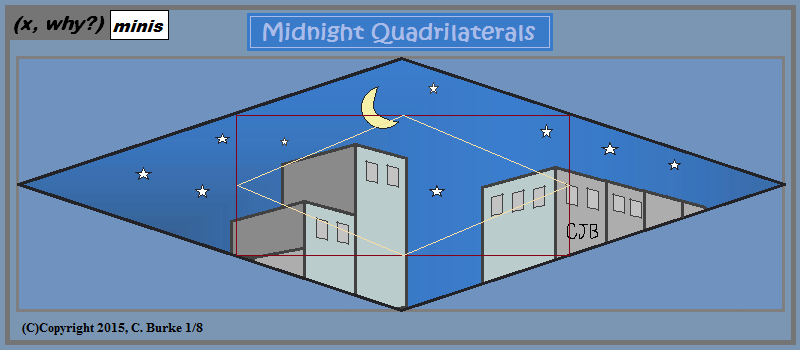It wasn't a typo. I just misread the title. I had hoped for a really cool article. Oh, well.

Fun things happen if you connect the midpoints of the sides of a quadrilateral, forming a new, smaller quadrilateral, and then connect those midpoints, and then those . . .Tuesday, January 06, 2015

Teacher Haiku, II

(Click on the comic if you can't see the full image.)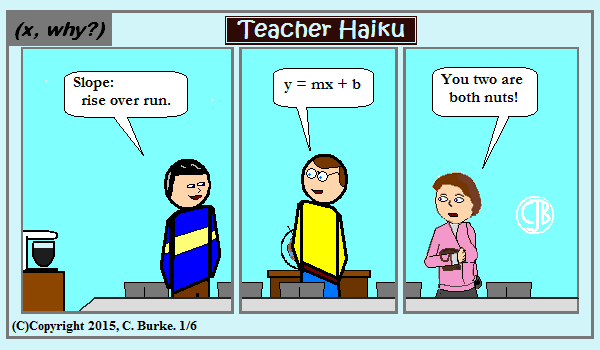Five more than seven / When increased another five / Totals seventeen.

You can translate that into an aritmetic expression, or you can consider it a shout-out to Jason Deeble's Monster Haiku, which as a daily M-F strip, is on track to pass the number of strips of this comic sometime in the next couple weeks.

Sure I probably could've timed it to find when they'd be equal -- solving a system of equations and all that -- but I'd probably forget to post one day and just throw it all off.

Also check out this haiku strip from March 2014.Monday, January 05, 2015

Those Daring Young Men

(Click on the comic if you can't see the full image.)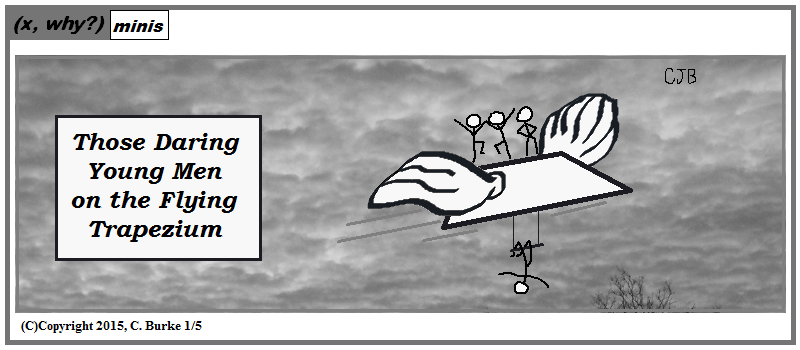Flying trapezoid just doesn't have the same ring.

I learned two things over the weekend. First is the word trapezium, which I don't recall encountering before. Outside of the U.S., it's a trapezoid. Apparently, inside the U.S., it's an irregular quadrilateral. That's probably the most interesting thing I've learned about trapezoids since college when a professor said that if the definition of trapezoid were at least one pair of parallel sides then parallelograms would be a special case of trapezoid, and all of it would fall under the Trapezoid Rule. (The triangular analog to this is that an equilateral traingle is a special type of isosceles triangle.)

The second thing I learned is that I have been mistaken for most of my life about a very old song that my parents would sing (and which was featured in at least one Popeye cartoon). The name of the song is The Daring Young Man of the Flying Trapeze. For whatever reason, possibly since the time I left kindergarten, I thought the lyrics were "those daring young men". It would make sense: you never see one acrobat; it's always a family or a troupe. Sure, one of them might be the "manly" one of the group to appeal to the young ladies, but to a five-year-old, who would notice? It's also possible that I've just been confusing it with the movie title Those Magnificent Men in Their Flying Machines, by which I don't mean Dick Dastardly and Muttley.

In any case, I already had four young men in the picture and I wasn't erasing three of them just to conform to an 80-year-old song.

Final notes: the background is a black-and-white rendition of a photo I took just after sunrise from my corner last week. The ones from my doorstep were better, but I went to the corner for one shot so I could avoid the traffic signal and lamppost.

The source of my initial sighting of "trapezium" was the cavmaths blog entry A Puzzling Heptagon.Sunday, January 04, 2015

Goals for 2015

"Looking at my list of goals for 2015 and I notice that the majority depend upon the acquisition of superpowers. Could prove an obstacle."

I do have a handful of goals -- more like suggestions, guidelines, really -- which will not require superpowers, but might involve managing or creating extra time. (Okay, so maybe that one is a superpower...)

A few things which I would like to do more of this year, in no particular order:

- Read more. Goal is a book a week, particularly now that I'm commuting by train instead of car. This is malleable -- I'm not going to stick to sixty-page novellas and avoid seven-hundred page monstrosities because I can't finish in time. And I reserve the right to use that week to evaluate if the book is even worth reading -- if I can't get through chapters 1 and 2 in four subway rides, it's not likely to happen. And, generally speaking, I usually hate 700-page books anyway.

-- Write more. Not just math articles, but stories, drafts of stories, whatever. I can't self-publish anything if there's nothing written in final form. I've taken too long off. Pick a plot and go for it. Maybe I'll troll twitter for plot suggestions, and ask for crazy combos. Whatever.

-- Record more. No, I'm not going to start a podcast, but I could start recording my blog entries in the same way that I recorded my class summaries a couple of years ago. I stopped because my students weren't listening to them. I know this because I hid Easter eggs in those reviews.

-- Transcribe. I used to keep writing journals. I have all of them. These days, I make notes in whatever's handy. I have three or four different notebooks with overlapping anecdotes and plot ideas. They need to be consolidated. Likewise, there are comic ideas buried everywhere, and I need to find them. Somewhere, I have the topic for this year's Mothers Day comic, and unless I find it again or the topic comes up again, I won't be making it this year.

In any case, the old journals should be typed up. I have twenty-year-old story ideas that need to be done already. Especially now that I have 20 years more writing experience and won't write stuff that will make me cringe when I re-read it. (At least, not as much.)

-- Learn how to make an eBook, and then make some eBooks. Put them on my kindle or put them on Amazon for sale. Whether it's an .epub fiction anthology or a .pdf collection of Common Core worksheets, I should have something out there. Branding.

-- Find a permanent teaching slot. It looks like I won't be going back to either of my last two teaching positions, so I need to find a new one where I'll be happy and less frustrated, where most of the students want to learn and the rest are able to once drawn to it. It's not out of the realm of possibilities.

-- Blog as I can. No, seriously, December taught me not to over-promise (particularly since I'm overestimating the number of people I'm actually promising). I delivered pretty well on the blogging, but the comic suffered. Even though the blog entries seem to get more hits than the comics, the comics are what got me started and they're my first love and first priority.